Data Structures and Algorithms with Object-Oriented Design Patterns in C## Exercises

1.   For each of the following key sequences determine the binary heap obtained when the keys are inserted one-by-one in the order given into an initially empty heap:
1. 0, 1, 2, 3, 4, 5, 6, 7, 8, 9.
2. 3, 1, 4, 1, 5, 9, 2, 6, 5, 4.
3. 2, 7, 1, 8, 2, 8, 1, 8, 2, 8.
2.   For each of the binary heaps obtained in Exercisedetermine the heap obtained after three consecutive DequeueMin operations.
3. Repeat Exercisesandfor a leftist heap.
4. Show the result obtained by inserting the keys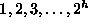one-by-one in the order given into an initially empty binomial queue.
5. A full binary tree is a tree in which each node is either a leaf or its is a full node (see Exercise). Consider a complete binary tree with n nodes.
1. For what values of n is a complete binary tree a full binary tree.
2. For what values of n is a complete binary a perfect binary tree.
6.   Prove by induction Theorem.
7. Devise an algorithm to determine whether a given binary tree is a heap. What is the running time of your algorithm?
8. Devise an algorithm to find the largest item in a binary min heap. Hint: First, show that the largest item must be in one of the leaves. What is the running time of your algorithm?
9.   Suppose we are given an arbitrary array of n keys to be inserted into a binary heap all at once. Devise an O(n) algorithm to do this. Hint: See Section.
10. Devise an algorithm to determine whether a given binary tree is a leftist tree. What is the running time of your algorithm?
11.   Prove that a complete binary tree is a leftist tree.
12. Suppose we are given an arbitrary array of n keys to be inserted into a leftist heap all at once. Devise an O(n) algorithm to do this. Hint: See Exercisesand.
13.   Consider a complete binary tree with its nodes numbered as shown in Figure. Let K be the number of a node in the tree. The the binary representation of K is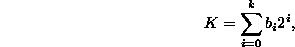where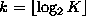.

1. Show that path from the root to a given node K passes passes through the following nodes: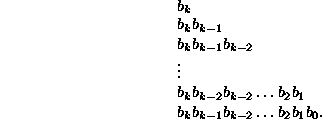2. Consider a complete binary tree with n nodes. The nodes on the path from the root to the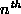are special. Show that every non-special node is the root of a perfect tree.
14. The Enqueue algorithm for the BinaryHeap class does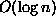object comparisons in the worst case. In effect, this algorithm does a linear search from a leaf to the root to find the point at which to insert a new key. Devise an algorithm that a binary search instead. Show that the number of comparisons required becomes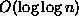. Hint: See Exercise.
15.   Prove Theorem.
16. Do Exercise.Copyright © 2001 by Bruno R. Preiss, P.Eng. All rights reserved.